# JP5021253B2 - Rotation angle detector - Google Patents

## Info

Publication number
JP5021253B2
JP5021253B2 JP2006227680A JP2006227680A JP5021253B2 JP 5021253 B2 JP5021253 B2 JP 5021253B2 JP 2006227680 A JP2006227680 A JP 2006227680A JP 2006227680 A JP2006227680 A JP 2006227680A JP 5021253 B2 JP5021253 B2 JP 5021253B2
Authority
JP
Japan
Prior art keywords
detection
magnetic
rotation angle
rotation
hall
Prior art date
Legal status (The legal status is an assumption and is not a legal conclusion. Google has not performed a legal analysis and makes no representation as to the accuracy of the status listed.)
Active
Application number
JP2006227680A
Other languages
Japanese (ja)
Other versions
JP2008051638A (en
Inventor

Original Assignee

Priority date (The priority date is an assumption and is not a legal conclusion. Google has not performed a legal analysis and makes no representation as to the accuracy of the date listed.)
Filing date
Publication date
Application filed by 株式会社デンソー, 株式会社日本自動車部品総合研究所 filed Critical 株式会社デンソー
Priority to JP2006227680A priority Critical patent/JP5021253B2/en
Publication of JP2008051638A publication Critical patent/JP2008051638A/en
Application granted granted Critical
Publication of JP5021253B2 publication Critical patent/JP5021253B2/en
Active legal-status Critical Current
Anticipated expiration legal-status Critical

• 238000004364 calculation methods Methods 0.000 claims description 26
• 239000004065 semiconductors Substances 0.000 claims description 7
• 230000004907 flux Effects 0.000 description 31
• 235000020127 ayran Nutrition 0.000 description 16
• 230000004323 axial length Effects 0.000 description 2
• 101000178139 fruit fly Protein dead ringer Proteins 0.000 description 2
• 238000004519 manufacturing process Methods 0.000 description 2
• 230000002093 peripheral Effects 0.000 description 2
• 230000003321 amplification Effects 0.000 description 1
• 230000000875 corresponding Effects 0.000 description 1
• 230000014509 gene expression Effects 0.000 description 1
• 238000003199 nucleic acid amplification method Methods 0.000 description 1
• 239000007787 solids Substances 0.000 description 1

## Images

•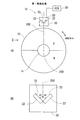•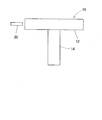•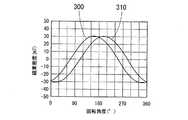••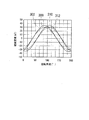•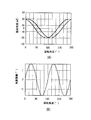•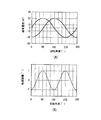•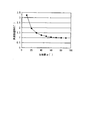•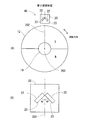•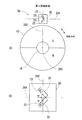•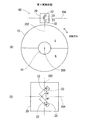•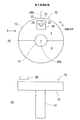•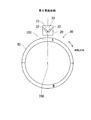•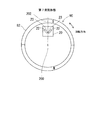•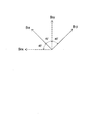•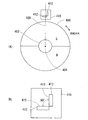•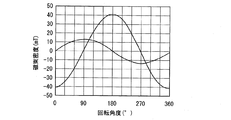•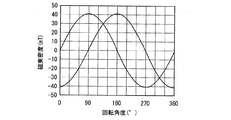•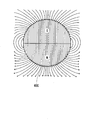## Classifications

• GPHYSICS
• G01MEASURING; TESTING
• G01DMEASURING NOT SPECIALLY ADAPTED FOR A SPECIFIC VARIABLE; ARRANGEMENTS FOR MEASURING TWO OR MORE VARIABLES NOT COVERED IN A SINGLE OTHER SUBCLASS; TARIFF METERING APPARATUS; MEASURING OR TESTING NOT OTHERWISE PROVIDED FOR
• G01D5/00Mechanical means for transferring the output of a sensing member; Means for converting the output of a sensing member to another variable where the form or nature of the sensing member does not constrain the means for converting; Transducers not specially adapted for a specific variable
• G01D5/12Mechanical means for transferring the output of a sensing member; Means for converting the output of a sensing member to another variable where the form or nature of the sensing member does not constrain the means for converting; Transducers not specially adapted for a specific variable using electric or magnetic means
• G01D5/14Mechanical means for transferring the output of a sensing member; Means for converting the output of a sensing member to another variable where the form or nature of the sensing member does not constrain the means for converting; Transducers not specially adapted for a specific variable using electric or magnetic means influencing the magnitude of a current or voltage
• G01D5/142Mechanical means for transferring the output of a sensing member; Means for converting the output of a sensing member to another variable where the form or nature of the sensing member does not constrain the means for converting; Transducers not specially adapted for a specific variable using electric or magnetic means influencing the magnitude of a current or voltage using Hall-effect devices
• G01D5/145Mechanical means for transferring the output of a sensing member; Means for converting the output of a sensing member to another variable where the form or nature of the sensing member does not constrain the means for converting; Transducers not specially adapted for a specific variable using electric or magnetic means influencing the magnitude of a current or voltage using Hall-effect devices influenced by the relative movement between the Hall device and magnetic fields

## Description

The present invention relates to a rotation angle detection device that detects a rotation angle of a detection target.

Conventionally, a rotation angle detection in which a magnet rotates with a detection target, a change in a magnetic field caused by the rotation of the magnet is detected by a magnetic detection element, and a rotation angle of the detection target is detected by trigonometric calculation based on an output signal of the magnetic detection element An apparatus is known (see, for example, Patent Document 1).
However, as in Patent Document 1, when the magnetic detection means is configured by installing a magnetic detection element in the vicinity of the circumference of the rotating magnet so as to be separated from the rotation direction, there is a problem that the entire size of the rotation angle detection device is enlarged. is there.

Further, in the configuration in which the magnetic detection elements are installed apart from each other in the rotation direction, the distance between each magnetic detection element and the magnet is likely to vary due to vibration or the like during or after assembly. Here, as shown in FIG. 19, since the magnetic flux density around the magnetic field generating means 400 formed of a permanent magnet or the like is not uniform over the entire circumference, the magnetic detection elements and the magnetic field generating means installed apart in the rotation direction. When the distance from 400 varies, the change rate of the magnetic flux density detected by each magnetic detection element is different, and the change rate of the output signal is also different. In addition, since the magnetic detection element usually has a temperature characteristic in which the output signal value changes according to the temperature, in the configuration in which the magnetic detection element is installed away from the rotation direction, if temperature distribution occurs in the rotation direction, The rate of change of the output signal of the magnetic detection element varies depending on the temperature. When the rotation angle is calculated by trigonometric function calculation from detection signals having different rates of change, there is a problem that an error in the calculated rotation angle increases.

Therefore, as shown in FIG. 16, it is conceivable to install the magnetic detection means 410 at one place in the rotation direction of the magnetic field generation means 400 to downsize the rotation angle detection device. One of the magnetic field generation means 400 or the magnetic detection means 410 rotates in conjunction with the detection target. In the magnetic detection means 410, for example, two Hall elements are used as the magnetic detection element 412. In FIG. 16, the magnetic sensing surface 413 of one of the two magnetic detection elements 412 is installed along a virtual straight line 420 orthogonal to the rotation axis 404 of the rotation shaft 402, and the other magnetic detection element 412 is disposed. The magnetosensitive surface 413 is set at 90 ° with respect to one of the magnetosensitive surfaces 413.

When two magnetic detection elements 412 are installed as shown in FIG. 16, the phase difference of the magnetic flux density detected by the two magnetic detection elements 412 becomes 90 ° according to the rotation angle as shown in FIG. . Since the magnetic detection element 412 outputs a signal corresponding to the magnetic flux density, the phase difference of the output signal of the magnetic detection element 412 is also 90 °.
However, as shown in FIG. 16, the magnetic sensing surface 413 of one magnetic detection element 412 is installed along a virtual straight line 420 orthogonal to the rotation axis 404 of the rotation shaft 402, and the magnetic detection surface of the other magnetic detection element 412 is arranged. When 413 is installed so as to form 90 ° with respect to one of the magnetic sensitive surfaces 413, as described above, the magnetic flux density around the magnetic field generating means 400 is not uniform over the entire circumference. A difference occurs in the magnitude of the magnetic flux density detected by the magnetic sensitive surface 413 of each magnetic detection element 412. That is, the amplitudes of the output signals of the two magnetic detection elements 412 are different. In order to detect the magnetic flux density that changes according to the rotation angle of the detection target with the magnetic detection element 412 and calculate the rotation angle by trigonometric function calculation from the output signal, the amplitude of the output signals of the two magnetic detection elements 412 Need to be equal.

Therefore, as shown in FIG. 18, it is conceivable to amplify the output with the smaller amplitude and match the amplitudes of the two output signals to reduce the error of the calculated rotation angle.
However, when the output signal is amplified, the error included in the original signal before amplification is amplified, and as a result, the calculated rotation angle error cannot be reduced. In addition, when an output signal having a small amplitude is read by an AD converter, a bit error increases, so that the rotation angle error cannot be reduced.

JP 2003-75108 A

The present invention has been made to solve the above problems, and an object of the present invention is to provide a rotation angle detection device that is small and has high detection accuracy of rotation angle.

According to the first to eighth aspects of the present invention, the magnetic detection means having the first magnetic detection element and the second magnetic detection element is provided at one place in the rotation direction so as to be rotatable relative to the magnetic field generation means. Therefore, the magnetic detection means can be downsized, and as a result, the rotation angle detection device can be downsized.
Furthermore, since the first magnetic detection element and the second magnetic detection element are installed at one place in the rotation direction, the operating environment of the magnetic detection element such as temperature changes to the same extent in both magnetic detection elements. Further, even if the distance between the magnetic detection means and the magnetic field generation means is deviated, the distance between the two magnetic detection elements and the magnetic field generation means is shifted to the same extent. As a result, even if the operating environment changes or the distance between the magnetic detection means and the magnetic field generation means shifts, the outputs of the two magnetic detection elements change to the same extent. Therefore, even if the operating environment changes or the distance between the magnetic detection means and the magnetic field generation means is shifted, the rotation angle of the detection target can be detected with high accuracy based on the output signals of the two magnetic detection elements.

Here, the maximum value of the magnetic flux density detected by the magnetosensitive surfaces 413 of the two magnetic detection elements 412 shown in FIG. 16 is set to two magnetic detection elements according to A and B, respectively, and the rotation angle θ of the magnetic field generating means 400. If the magnetic flux densities detected by 412 are B 0 x and B 0 y, respectively, B 0 x and B 0 y are expressed by the following equations (1) and (2). Since the two magnetic detection elements 412 output signals obtained by multiplying the values of the detected magnetic flux densities B 0 x and B 0 y by coefficients, the detected magnetic flux densities B 0 x and B 0 y may be regarded as output signals. Hereinafter, B k x and B k y (k = 0, 1, 2,...) May be referred to as outputs.
B 0 x = A × sin θ (1)
B 0 y = −B × cos θ (2)

Next, in the invention described in claims 1 to 8, on the virtual plane orthogonal to the rotation axis, the direction in which the magnetic sensitive surface of the first magnetic detection element extends and the direction in which the magnetic sensitive surface of the second magnetic detection element extends. Are installed so as to form 90 °, and the magnetic sensing elements installed so that the extending directions of both magnetosensitive surfaces are inclined by 45 ° with respect to a virtual straight line orthogonal to the rotation axis are rotated relative to the magnetic detection means. Assuming that the magnetic flux densities detected in accordance with the rotation angle θ of the magnetic field generating means to perform are B 1 x and B 1 y (see FIG. 15), B 1 x and B 1 y are expressed by the following equation (3) by combining the vectors. (4).

B 1 x = B 0 x × cos 45 + B 0 y × sin 45 (3)
B 1 y = −B 0 x × sin 45 + B 0 y × cos 45 (4)
From formulas (1) to (4),
B 1 x = B 0 x / (2 1/2 ) + B 0 y / (2 1/2 )
= A × sin θ / (2 1/2 ) −B × cos θ / (2 1/2 )
= ((A 2 + B 2 ) / 2) 1/2 × sin (θ + β) (5)
B 1 y = −B 0 x / (2 1/2 ) + B 0 y / (2 1/2 )
= −A × sin θ / (2 1/2 ) −B × cos θ / (2 1/2 )
= ((A 2 + B 2 ) / 2) 1/2 × sin (θ + γ) (6)

Equation (5), as can be seen from (6), in the configuration shown in FIG. 16 described above, two maximum value A of the detected magnetic flux density of the magnetic sensor 412, even if different B, and claim 1 8 In the invention described in ( 2 ), the maximum value of the magnetic flux density detected by the two magnetic detection elements is ((A 2 + B 2 ) / 2) 1/2 and is equal. That is, the amplitudes of the output signals of the two magnetic detection elements are equal.
This eliminates the need to amplify the output value of one of the two magnetic detection elements having different amplitudes in accordance with the output value of the other, so that the rotation angle of the detection target can be easily and accurately detected. Can be detected.

According to the second aspect of the present invention, the magnetic field generating means is formed of a disk-shaped or cylindrical permanent magnet. Therefore, when the permanent magnet rotates relative to the magnetic detection element, the magnetic field generating means depends on the rotation angle. The waveform of the detection signal output from the magnetic detection element can be made closer to a sine waveform. Therefore, the rotation angle can be detected with high accuracy from the output signals of the two magnetic detection elements.

According to the third aspect of the present invention, since the two magnetic detection elements are formed of one chip of semiconductor, for example, two magnetic detection elements are magnetically formed in the semiconductor manufacturing process rather than formed of separate chips. The angle formed by the detection element can be 90 ° with high accuracy. Furthermore, the operating environments of the two magnetic detection elements match. Therefore, the rotation angle can be detected with high accuracy.

Here, from equation (5)
sin β = −B / (A 2 + B 2 ) 1/2 (7)
cos β = A / (A 2 + B 2 ) 1/2 (8)
Therefore,
β = cos −1 (A / (A 2 + B 2 ) 1/2 ) (9)
From equation (6),
sin γ = −B / (A 2 + B 2 ) 1/2 (10)
cosγ = −A / (A 2 + B 2 ) 1/2 (11)
Therefore,
γ = cos −1 (−A / (A 2 + B 2 ) 1/2 )
= 180 ° -β (12)
Therefore, the phase difference (β−γ) of the output signals of the two magnetic detection elements is expressed by the following equation (13).
(Β−γ) = β− (180 ° −β) = 2 × (β−90 °) (13)

In the first aspect of the present invention, the two magnetic detection elements are installed so that the magnetosensitive surface forms 90 °, but as can be seen from the equation (13), the outputs of the two magnetic detection elements. The phase difference (β−γ) of the signal is shifted from 90 °. As can be seen from the equations (9) and (12), the phase difference (β−γ) is equal to the maximum values A and B of the magnetic flux density detected by the two magnetic detection elements 412 in the configuration shown in FIG. Will change accordingly.

Here, in the configuration shown in FIG. 16, when the position of the magnetic detection means 410 is displaced in the radial direction with respect to the magnetic field generation means 400, the maximum values A and B of the magnetic flux density detected by the two magnetic detection elements 412 change. To do. Therefore, in the first aspect of the present invention, when the position of the magnetic detection means, that is, the magnetic detection element is shifted in the radial direction with respect to the magnetic field generation means, the equations (5), (6), (9), (12 ) Change in the values of A and B, the amplitude and phase of the output of the magnetic detection element change. However, in the invention described in claim 1, as can be seen from the equations (5) and (6), the position of the magnetic detection element is shifted in the radial direction with respect to the magnetic field generating means, and the outputs of the two magnetic detection elements are output. Even if the amplitude changes, both amplitudes are multiplied by a constant to be equal. Therefore, even if the amplitude changes, an error in the rotation angle does not occur. However, in the first aspect of the invention, as can be seen from the equation (13), when the output phases of the two magnetic detection elements change, the output phase difference changes. When the phase difference changes, an error occurs in the rotation angle calculated by trigonometric function calculation. Here, it is known that the rotation angle error caused by the change in the phase difference is the smallest when the phase difference of the output of the magnetic detection element is 90 °.

Therefore, in the invention according to claim 5 , since the calculation means calculates the rotation angle of the detection target by correcting the phase difference between the two output signals to 90 °, the position of the magnetic detection element is a diameter relative to the magnetic field generation means. Even if the direction is deviated, the error of the rotation angle calculated by the calculating means can be minimized.
In the fifth aspect of the invention, by correcting the phase difference between the two output signals to 90 °, the two output signals have a relationship of sin and cos. Accordingly, assuming that the values of both output signals with the phase difference corrected to 90 ° are Bx and By and the rotation angle of the magnetic field generating means is θ, for example, by a simple trigonometric function calculation of θ = tan −1 (Bx / By) The rotation angle of the detection target can be easily calculated.

A plurality of embodiments of the present invention will be described with reference to the drawings.
(First embodiment)
A rotation angle detection device according to a first embodiment of the present invention is shown in FIG. The rotation angle detection device 10 is a device that detects a rotation angle of a detection target such as a crankshaft or a wheel. The rotation angle detection apparatus 10 includes a disk-shaped permanent magnet 12, a Hall IC 20 as an angle sensor, and an electronic control unit (ECU) 30. The permanent magnet 12 as the magnetic field generating means rotates around the rotation shaft 14 in conjunction with the detection target. The permanent magnet 12 is formed in a disk shape and is magnetized in the radial direction. The permanent magnet 12 forms a magnetic field shown in FIG.

The Hall IC 20 as the magnetic detection means is installed at one place in the rotation direction of the permanent magnet 12. Thereby, the rotation angle detection apparatus 10 can be reduced in size. The Hall IC 20 is an angle sensor in which two Hall elements 22 are mounted as a one-chip semiconductor. From the Hall IC 20, detection signals of the two Hall elements 22 are respectively output to the ECU 30. In the rotation angle detection device 10, the two Hall elements 22 and the ECU 30 may be separate components, or may be configured as a single chip as a single semiconductor. As shown in FIGS. 1 and 2, the Hall IC 20 is disposed on the outer side in the radial direction than the permanent magnet 12 so as to face the outer peripheral surface of the permanent magnet 12.

The two Hall elements 22 each have a magnetosensitive surface 23 with respect to the virtual straight line 202 on both sides of the virtual straight line 202 orthogonal to the rotation axis 200 on a virtual plane orthogonal to the rotation axis 200 of the rotation shaft 14. It is inclined at an angle of 45 ° and is installed so that the crossing angle of the two magnetosensitive surfaces 23 is 90 °.
The ECU 30 serving as calculation means includes a CPU, ROM, RAM, EEPROM, etc. (not shown). ECU30 calculates the rotation angle of a detection target from the output signal of Hall IC20 by running the angle calculation program memorize | stored in EEPROM or ROM.

Next, a method for calculating the rotation angle of the detection target by the rotation angle detection device 10 will be described.
(Calculation method 1)
As described above, the amplitudes of the magnetic flux densities 300 and 310 detected by the two Hall elements 22 are equal as shown in FIG. 3 from the expressions (5) and (6). When the maximum amplitude is ((A 2 + B 2 ) / 2) 1/2 = V, from the equations (5), (6) and (12),
B 1 x = V × sin (θ + β) (20)
B 1 y = V × sin (θ + γ)
= V × sin (θ + (180 ° −β))
= −V × sin (θ−β) (21)
here,
B 1 x + B 1 y = V × sin (θ + β) −V × sin (θ−β)
= 2V × cos θ sin β (22)
B 1 x−B 1 y = V × sin (θ + β) + V × sin (θ−β)
= 2V × sin θ cos β (23)

From equations (22) and (23),
(B 1 x−B 1 y) / (B 1 x + B 1 y) = sin θ cos β / cos θ sin β
= Tan θcotβ
Therefore,
θ = tan −1 (tan β × (B 1 x−B 1 y) / (B 1 x + B 1 y)) (24)
From the equation (24), in the first embodiment shown in FIG. 1, the phase angle β of one output B 1 x of the two Hall elements 22 and the outputs B 1 x and B 1 y of the two Hall elements 22 are obtained. Thus, the rotation angle θ of the detection target can be calculated by trigonometric function calculation as shown in FIG.

(Calculation method 2)
If the maximum values A and B of the magnetic flux density detected by each Hall element are measured in advance when the Hall element is installed as shown in FIG. 16, tan β = (− B / A), from equation (24),
θ = tan −1 (− (B / A) × (B 1 x−B 1 y) / (B 1 x + B 1 y))
= −tan −1 ((B / A) × (B 1 x−B 1 y) / (B 1 x + B 1 y))
... (30)
It is. Accordingly, when the Hall elements are installed as shown in FIG. 16, if the maximum values A and B of the magnetic flux density detected by each Hall element are measured in advance, the maximum values A and B of the detected magnetic flux density From the outputs B 1 x and B 1 y of the two Hall elements 22 of this embodiment installed as shown in FIG. 1, the rotation angle θ to be detected can be calculated by trigonometric function calculation. The maximum values A and B are actually the maximum values of the output of each Hall element when the Hall element is installed as shown in FIG.

(Calculation method 3)
Since tan β = cot (90 ° −β), from the equation (24),
θ = tan −1 (cot (90 ° −β) × (B 1 x−B 1 y) / (B 1 x + B 1 y))
... (40)
Here, if the phase difference between the outputs of the two Hall elements 22 is α,
α = β−γ = β− (180 ° −β) = − (180 ° −2β)
Therefore,
-Α / 2 = 90 ° -β (41)
Therefore, from the equations (40) and (41),
θ = tan −1 (cot (−α / 2) × (B 1 x−B 1 y) / (B 1 x + B 1 y))
= −tan −1 (cot (α / 2) × (B 1 x−B 1 y) / (B 1 x + B 1 y))
... (42)

Therefore, from the phase difference α between the two Hall elements 22 and the outputs B 1 x and B 1 y of the two Hall elements 22, the rotation angle θ to be detected can be calculated by trigonometric function calculation.
Any one of the calculation methods 1, 2, and 3 described above is selected and the rotation angle of the detection target is calculated. In calculation method 4 described below, the rotation angle is calculated in combination with calculation methods 1, 2, and 3.

(Calculation method 4)
When the position of the Hall IC 20 is shifted in the radial direction with respect to the permanent magnet 12, as described above, the amplitude of the output signals 300 and 310 of the Hall element 22 and the phase of the output signals 300 and 310 change (see FIG. 5). . However, the amplitudes of the output signals 300 and 310 of the Hall element 22 are both output signals 302 and 312 that are multiplied by a constant. Therefore, the change in amplitude does not affect the calculation result of the angle. However, when the position of the Hall IC 20 is shifted in the radial direction with respect to the permanent magnet 12, the phase of the output signals 300 and 310 of the Hall element 22 changes as shown in FIG. The phase difference from the signal 312 changes. Thus, when the phase difference between the output signals of the two Hall elements 22 changes, an error occurs in the rotation angle calculated from the output signals of the two Hall elements 22.

FIG. 8 shows the relationship between the phase difference between the output signals of the two Hall elements 22 and the calculated rotation angle error. It can be seen that the angle error decreases as the phase difference increases toward 90 °. For example, the relationship between the detection signal when the phase difference is smaller than 90 ° and the error of the rotation angle calculated from the detection signal is shown in FIG. 6, and the detection signal when the phase difference is 90 ° and the detection signal FIG. 7 shows the relationship with the rotation angle error calculated from FIG. In FIG. 6A and FIG. 7A, the solid line indicates the detection signal before the positional deviation, and the dotted line indicates the detection signal after the positional deviation. The angle error is smaller when the phase difference is 90 °.

Therefore, based on the values of the output signals of the two Hall elements 22, the map is referred to, and the values of the output signals of the two Hall elements 22 are corrected so that the phase difference is 90 °. Then, the rotation angle is calculated from the output signal of the Hall element 22 after the phase difference is corrected to 90 °. The outputs of the two Hall elements 22 with the phase difference corrected to 90 ° have a relationship between sin and cos having the same amplitude. Therefore, if the values of both output signals with the phase difference corrected to 90 ° are Bx and By, for example, the rotation angle of the detection target can be easily determined by simple trigonometric calculation of θ = tan −1 (Bx / By). It can be calculated.
Thus, even if the position of the Hall IC 20 is shifted in the radial direction with respect to the permanent magnet 12, the error of the calculated rotation angle is corrected by correcting the phase difference between the outputs of the two Hall elements 22 to 90 °. The rotation angle of the detection target can be easily calculated while making it as small as possible.

(Temperature characteristic compensation)
Next, calculation of the rotation angle when temperature characteristics are taken into account in the first embodiment will be described.
In equations (1) and (2), the detected magnetic flux density when the temperature characteristic is taken into consideration is B 2 x and B 2 y, respectively, and the detected magnetic flux density when the degree characteristic is taken into consideration in equations (5) and (6), respectively. The phase angles of the output signals of the two Hall elements 22 when the temperature characteristics are taken into account in B 3 x, B 3 y, Equations (5) and (6), respectively, are β 3 , γ 3 , and the temperature characteristics of the Hall elements 22 When the coefficient is k (t), B 2 x, B 2 y, B 3 x, and B 3 y are expressed by the following equations (50), (51), (52), and (53).

B 2 x = k (t) × B 0 x = A × k (t) × sin θ (50)
B 2 y = k (t) × B 0 y = −B × k (t) × cos θ (51)
B 3 x = B 2 x / (2 1/2 ) + B 2 y / (2 1/2 )
= A × k (t) × sin θ / (2 1/2 ) −B × k (t) × cos θ / (2 1/2 )
= K (t) × ((A 2 + B 2 ) / 2) 1/2 × sin (θ + β 3 )
... (52)
B 3 y = −B 2 x / (2 1/2 ) + B 2 y / (2 1/2 )
= −A × k (t) × sin θ / (2 1/2 ) −B × k (t) × cos θ / (2 1/2 )
= K (t) × ((A 2 + B 2 ) / 2) 1/2 × sin (θ + γ 3 )
... (53)

Here, from equation (52)
cos β 3 = A / (A 2 + B 2 ) 1/2
Therefore, from the equations (8) and (9),
β 3 = cos −1 (A / (A 2 + B 2 ) 1/2 ) = β (54)
From the equation (53),
cos γ 3 = −A / (A 2 + B 2 ) 1/2
Therefore, from formulas (11) and (12), γ 3 = cos −1 (−A / (A 2 + B 2 ) 1/2 )
= Γ = 180 ° −β (55)

From equations (52) to (55), it can be seen that the phase angle and phase difference of the output signals of the two Hall elements 22 do not change even when the temperature changes, and the amplitude is multiplied by k (t). And from the equations (5), (6), (52) to (55),
B 3 x = k (t) × ((A 2 + B 2 ) / 2) 1/2 × sin (θ + β 3 )
= K (t) × B 1 x (56)
B 3 y = k (t) × ((A 2 + B 2 ) / 2) 1/2 × sin (θ + γ 3 )
= K (t) × B 1 y (57)

In equation (24), when B 1 x is replaced with B 3 x, B 1 y is replaced with B 3 y, and equations (56) and (57) are substituted,
θ = tan −1 (tan β × (B 3 x−B 3 y) / (B 3 x + B 3 y))
= Tan −1 (tan β × k (t) × (B 1 x−B 1 y) / (k (t) × (B 1 x + B 1 y)))
= Tan −1 (tan β × (B 1 x−B 1 y) / (B 1 x + B 1 y)) (58)
That is, in this embodiment, even if the ambient temperature changes, the calculation result of the rotation angle is not affected by the temperature change. Therefore, the rotation angle can be detected with high accuracy regardless of the change in the ambient temperature.

(Second to seventh embodiments)
Second to seventh embodiments of the present invention are shown in FIGS. 9 to 14. 9 to 14, the ECU 30 is omitted. In addition, the same code | symbol is attached | subjected to substantially the same component as embodiment mentioned above.
Also in the second to seventh embodiments, the magnetosensitive surfaces 23 of the two Hall elements 22 are installed so that the crossing angle is 90 ° on a virtual plane orthogonal to the rotation axis 200 of the rotation shaft 14. Yes. Further, the magnetosensitive surface 23 is inclined at an angle of 45 ° with respect to the virtual straight line 202 orthogonal to the rotation axis 200.

In the rotation angle detection device 40 of the second embodiment shown in FIG. 9, the two Hall elements 22 are arranged on a virtual plane orthogonal to the rotation axis 200 of the rotation shaft 14 in the same manner as in the first embodiment. The magnetosensitive surface 23 is inclined at an angle of 45 ° with respect to the virtual straight line 202 on both sides of the virtual straight line 202 orthogonal to each other, and the crossing angle of the two magnetosensitive surfaces 23 is 90 °. Has been. However, the rotation angle detection device 40 is installed by rotating the hole 1C20 of the first embodiment shown in FIG. 1 by 180 °. That is, in the rotation angle detection device 40, the magnetosensitive surfaces 23 of the two Hall elements 22 are inclined at an angle of 45 ° on both sides across the virtual straight line 202 to form a crossing angle of 90 °, and the permanent magnet Open to 12.

In the rotation angle detection devices 50 and 60 of the third and fourth embodiments shown in FIGS. 10 and 11, the two Hall elements 22 are arranged on the rotation axis 200 on a virtual plane orthogonal to the rotation axis 200 of the rotation shaft 14. The magnetosensitive surface 23 is inclined at an angle of 45 ° with respect to the imaginary straight line 202 orthogonal to each other, and the crossing angle of the two magnetosensitive surfaces 23 is 90 °. However, the rotation angle detection devices 50 and 60 are installed by rotating the hall 1C20 of the first embodiment shown in FIG. That is, in the rotation angle detection devices 50 and 60, the magnetic sensing surfaces 23 of the two Hall elements 22 are on both sides of the virtual straight line 204 along the tangent line of the disk-shaped permanent magnet 12, with respect to the virtual straight line 204. Each of them is inclined at an angle of 45 ° to form a crossing angle of 90 ° and is open toward one of the tangential directions of the permanent magnet 12.

In the rotation angle detection device 70 of the fifth embodiment shown in FIG. 12, the Hall IC 20 is installed on the end surface on the outer peripheral side of the permanent magnet 12.
In the rotation angle detector 80 of the sixth embodiment shown in FIG. 13, the permanent magnet 82 as the magnetic field generating means is formed in an annular shape or a cylindrical shape. The Hall IC 20 is disposed on the outer side in the radial direction of the permanent magnet 82 so as to face the permanent magnet 82 within the range of the axial length of the permanent magnet 82.
In the rotation angle detection device 90 of the seventh embodiment shown in FIG. 14, the Hall IC 20 is installed on the radially inner side of the permanent magnet 82 so as to face the permanent magnet 82 within the range of the axial length of the permanent magnet 82. Yes.

(Other embodiments)
In the embodiment described above, the permanent magnet as the magnetic field generating means rotates in conjunction with the detection target. On the other hand, the Hall IC as the magnetic detection means may rotate in conjunction with the detection target.
In the above embodiment, an electromagnet or the like may be used as the magnetic field generating means.
In the above embodiment, the Hall IC 20 is formed by manufacturing two Hall elements as a one-chip semiconductor. On the other hand, two Hall elements are manufactured as semiconductors of different chips, the magnetosensitive surface forms 90 °, and the magnetosensitive surface is inclined at an angle of 45 ° with respect to a virtual straight line orthogonal to the rotation axis. Two Hall elements may be installed in the.

As in this, the present invention is not limited to the above plurality of embodiments are applicable to various embodiments within a scope not departing from the gist.

(A) is a top view which shows the rotation angle detection apparatus by 1st Embodiment, (B) is an enlarged view of Hall IC. The II direction arrow directional view of FIG. The characteristic view which shows the relationship between a rotation angle and the detected magnetic flux density of two Hall elements. It is a characteristic view which shows the relationship between a rotation angle and a calculation angle. It is a characteristic view which shows the change of the detected magnetic flux density by position shift. (A) shows the relationship between the detected magnetic flux density before and after misalignment with respect to the rotational angle before phase correction, and (B) shows the error between the rotational angle and the angle error calculated from the detected magnetic flux density due to misalignment. The characteristic view which shows a relationship. (A) shows the relationship between the detected magnetic flux density before and after misalignment with respect to the rotation angle after phase correction, and (B) shows the error between the rotation angle and the angle error calculated from the detected magnetic flux density due to misalignment. The characteristic view which shows a relationship. The characteristic view which shows the relationship between a phase difference and an angle error. (A) is a top view which shows the rotation angle detection apparatus by 2nd Embodiment, (B) is an enlarged view of Hall IC. (A) is a top view which shows the rotation angle detection apparatus by 3rd Embodiment, (B) is an enlarged view of Hall IC. (A) is a top view which shows the rotation angle detection apparatus by 4th Embodiment, (B) is an enlarged view of Hall IC. (A) is a top view which shows the rotation angle detection apparatus by 5th Embodiment, (B) is a B direction arrow directional view of (A). The top view which shows the rotation angle detection apparatus by 6th Embodiment. The top view which shows the rotation angle detection apparatus by 7th Embodiment. Explanatory drawing which shows the magnetic flux detection direction of the magnetic detection element of the past and this invention. (A) is a top view which shows the conventional rotation angle detection apparatus, (B) is an enlarged view of a magnetic detection means. The characteristic view which shows the relationship between the rotation angle in FIG. 16, and the detection magnetic flux density of a magnetic detection means. The characteristic view which shows the relationship between the rotation angle which matched the amplitude, and the detection magnetic flux density of a magnetic detection means. Explanatory drawing of the magnetic field formed around a magnetic field generation means.

Explanation of symbols

10, 40, 50, 60, 70, 80, 90: Rotation angle detection device, 12, 82: Permanent magnet (magnetic field generation means), 20: Hall IC, (magnetic detection means), 22: Hall element (magnetic detection element) ), 30: ECU (calculation means), 200: rotation axis, 202: virtual straight line

## Claims (8)

1. In the rotation angle detection device for detecting the rotation angle of the detection target,
Magnetic field generating means;
Magnetic detection means that rotates relative to the magnetic field generation means as the detection object rotates;
With
The magnetic detection means is installed at one place in the rotation direction so as to be rotatable relative to the magnetic field generation means, and responds to a change in magnetic field generated by relative rotation of the magnetic detection means with respect to the magnetic field generation means. A first magnetic detection element and a second magnetic detection element for outputting a signal;
The two magnetic detection elements are Hall elements, and on the virtual plane orthogonal to the rotation axis of relative rotation, the direction in which the magnetic sensing surface of the first magnetic detection element extends and the magnetic sensing surface of the second magnetic detection element are The rotation angle detecting device is installed so that the extending direction forms 90 °, and the extending directions of the two magnetosensitive surfaces are inclined by 45 ° with respect to a virtual straight line orthogonal to the rotation axis.
2.   The rotation angle detecting device according to claim 1, wherein the magnetic field generating means is a disc-shaped or cylindrical magnet.
3. The rotation angle detection device according to claim 1 or 2 , wherein the first magnetic detection element and the second magnetic detection element are formed of a single chip semiconductor.
4. Based on the second output signal of the first output signal and said second magnetic detecting element of the first magnetic detection element, the claim 1, further comprising calculating means for calculating a rotation angle of the detection target by trigonometric function operation 3 The rotation angle detection device according to any one of the above.
5. The rotation angle detection device according to claim 4 , wherein the calculation unit calculates a rotation angle of the detection target by correcting a phase difference between the two output signals to 90 °.
6. The rotation angle detection device according to claim 4 , wherein the calculation unit calculates the rotation angle of the detection target from one phase angle of the both output signals with respect to the rotation angle of the detection target and the both output signals.
7. The rotation angle detection apparatus according to claim 4 , wherein the calculation unit calculates a rotation angle of the detection target from a phase difference between the output signals and the output signals.
8. On one virtual plane perpendicular to the rotation axis, one magnetic sensing surface of two magnetic sensing elements is installed along a virtual straight line perpendicular to the rotational axis, and the other magnetic sensing element is placed on the one magnetic sensing element. When the 90.degree. Surface is installed, the calculating means calculates the detection value from the maximum value of the output signals of the both magnetic detection elements temporarily installed, the first output signal, and the second output signal. The rotation angle detection device according to claim 4 , wherein the rotation angle of the object is calculated.
JP2006227680A 2006-08-24 2006-08-24 Rotation angle detector Active JP5021253B2 (en)

## Priority Applications (1)

Application Number Priority Date Filing Date Title
JP2006227680A JP5021253B2 (en) 2006-08-24 2006-08-24 Rotation angle detector

## Applications Claiming Priority (3)

Application Number Priority Date Filing Date Title
JP2006227680A JP5021253B2 (en) 2006-08-24 2006-08-24 Rotation angle detector
US11/878,523 US7663360B2 (en) 2006-08-24 2007-07-25 Rotation angle detecting device
DE200710000440 DE102007000440A1 (en) 2006-08-24 2007-08-13 Rotational angle detection device comprises magnetic element for producing magnetic field and magnetic sensor with pair of sensor elements for detecting change in magnetic field

## Publications (2)

Publication Number Publication Date
JP2008051638A JP2008051638A (en) 2008-03-06
JP5021253B2 true JP5021253B2 (en) 2012-09-05

# Family

## Family Applications (1)

Application Number Title Priority Date Filing Date
JP2006227680A Active JP5021253B2 (en) 2006-08-24 2006-08-24 Rotation angle detector

## Country Status (3)

US (1) US7663360B2 (en)
JP (1) JP5021253B2 (en)
DE (1) DE102007000440A1 (en)

## Families Citing this family (17)

* Cited by examiner, † Cited by third party
Publication number Priority date Publication date Assignee Title
US7469604B2 (en) * 2005-10-21 2008-12-30 Stoneridge Control Devices, Inc. Sensor system including a magnetized shaft
JP2009058240A (en) * 2007-08-30 2009-03-19 Denso Corp Rotation detector
JP5192926B2 (en) * 2008-06-30 2013-05-08 古河電気工業株式会社 Rotation angle detector
US7830216B1 (en) * 2008-09-23 2010-11-09 Silicon Labs Sc, Inc. Precision, temperature stable clock using a frequency-control circuit and a single oscillator
JP5879025B2 (en) * 2010-03-26 2016-03-08 旭化成エレクトロニクス株式会社 Hall element
JP5105263B2 (en) * 2010-03-29 2012-12-26 株式会社デンソー Rotation angle detector
CN102022974B (en) * 2010-10-29 2013-01-23 昌辉汽车电气系统（安徽）有限公司 Calibration method of magnetic induction effect angle sensor
US9062989B2 (en) * 2010-12-15 2015-06-23 Nxp B.V. Magnetic field sensor for sensing rotation a reference component about the axis of rotation that is independent of alignment between the axis of rotation and the sensors
DE102011008614A1 (en) * 2010-12-30 2012-07-05 Siemens Aktiengesellschaft Determining the angular position of a rotor
JP5141780B2 (en) * 2011-01-12 2013-02-13 Ｔｄｋ株式会社 Rotation angle sensor
DE202011002402U1 (en) 2011-02-04 2012-05-07 Dr. Fritz Faulhaber Gmbh & Co. Kg Electric micromotor
EP2485374B2 (en) 2011-02-04 2017-03-01 Dr. Fritz Faulhaber GmbH & Co. KG Miniature electric motor
JP5692607B2 (en) * 2012-07-05 2015-04-01 株式会社デンソー Position detecting device and manufacturing method thereof
CN103915233B (en) * 2013-01-05 2017-02-08 江苏多维科技有限公司 Permanent magnet suitable for magnetic angle encoder
WO2016013650A1 (en) * 2014-07-25 2016-01-28 三菱電機株式会社 Magnetic sensor device
US9933448B1 (en) 2017-01-11 2018-04-03 Infineon Technologies Ag Stray-field robust, twist-insensitive magnetic speed sensors
DE102017109531B3 (en) 2017-05-04 2018-07-19 Schaeffler Technologies AG & Co. KG Sensor arrangement and method for generating a position change signal sequence

## Family Cites Families (10)

* Cited by examiner, † Cited by third party
Publication number Priority date Publication date Assignee Title
JPS6276607U (en) * 1985-10-31 1987-05-16
JPH0420812A (en) * 1990-05-15 1992-01-24 Tokai Rika Co Ltd Rotation angle detector
US5644226A (en) * 1994-03-02 1997-07-01 Nippondenso Co., Ltd. Magnetic detector having a bias magnet and magnetoresistive elements shifted away from the center of the magnet
JP2982638B2 (en) * 1995-01-19 1999-11-29 株式会社デンソー Displacement detector
US6326781B1 (en) * 1999-01-11 2001-12-04 Bvr Aero Precision Corp 360 degree shaft angle sensing and remote indicating system using a two-axis magnetoresistive microcircuit
JP2003004412A (en) * 2001-06-21 2003-01-08 Tokai Rika Co Ltd Rotary angle detector
JP2003075108A (en) 2001-09-04 2003-03-12 Asahi Kasei Corp Rotation angle sensor
JP4376150B2 (en) 2004-08-06 2009-12-02 株式会社デンソー Rotation angle detector
JP4679358B2 (en) 2005-02-03 2011-04-27 株式会社デンソー Rotation angle detector
JP4519750B2 (en) 2005-09-29 2010-08-04 株式会社デンソー Rotation angle detector

## Also Published As

Publication number Publication date
US7663360B2 (en) 2010-02-16
US20080048651A1 (en) 2008-02-28
DE102007000440A1 (en) 2008-02-28
JP2008051638A (en) 2008-03-06

## Similar Documents

Publication Publication Date Title
EP2769192B1 (en) Magneto-elastic torsion or torque sensor and method for determining torsion or torque using such a sensor
US9810551B2 (en) On-axis magnetic field angle sensors, systems and method
JP5834292B2 (en) Current sensor
JP5450948B2 (en) Wheel bearing with rotation detector
CA2612932C (en) Absolute encoder and method for generating an absolute value for an angle of rotation
US8587295B2 (en) Angle detection apparatus and position detection apparatus
US8319493B2 (en) Rotation detecting device and bearing assembly equipped with such rotation detecting device
US8490499B2 (en) Sensor system for ascertaining a torque and for index detection
EP1417496B1 (en) Tachometer apparatus and method for motor velocity measurement
EP2338030B2 (en) Magnetic position sensor with field direction measurement and flux collector
US7202659B2 (en) Rotation angle-detecting device
US6661225B2 (en) Revolution detecting device
JP4002174B2 (en) Magnetic rudder angle detector
US8664943B2 (en) Position detecting apparatus
US7030608B2 (en) Rotational angle sensing device and assembling method thereof
US7583080B2 (en) Rotation angle detection device and rotation angle correction method
JP2010078366A (en) Angle detecting apparatus
EP3084456B1 (en) Magnetic field sensor and method for sensing relative location of the magnetic field sensor and a target object along a movement line
KR20150039213A (en) Angular or linear magnetic position sensor not sensitive to external fields
JP5666886B2 (en) Rotary encoder
US9200924B2 (en) Magnetic out-of-axis angle sensing principle
EP2244070A1 (en) Rotation angle detection device, rotary machine, and rotation angle detection method
US6791217B2 (en) Method and system for motor velocity measurement
US7319320B2 (en) Rotation angle detecting device
JP6043721B2 (en) Improved position sensor

## Legal Events

Date Code Title Description
A621 Written request for application examination

Free format text: JAPANESE INTERMEDIATE CODE: A621

Effective date: 20081015

A977 Report on retrieval

Free format text: JAPANESE INTERMEDIATE CODE: A971007

Effective date: 20101122

A131 Notification of reasons for refusal

Free format text: JAPANESE INTERMEDIATE CODE: A131

Effective date: 20110106

A521 Written amendment

Free format text: JAPANESE INTERMEDIATE CODE: A523

Effective date: 20110218

A131 Notification of reasons for refusal

Free format text: JAPANESE INTERMEDIATE CODE: A131

Effective date: 20120323

A521 Written amendment

Free format text: JAPANESE INTERMEDIATE CODE: A523

Effective date: 20120508

TRDD Decision of grant or rejection written
A01 Written decision to grant a patent or to grant a registration (utility model)

Free format text: JAPANESE INTERMEDIATE CODE: A01

Effective date: 20120608

A01 Written decision to grant a patent or to grant a registration (utility model)

Free format text: JAPANESE INTERMEDIATE CODE: A01

A61 First payment of annual fees (during grant procedure)

Free format text: JAPANESE INTERMEDIATE CODE: A61

Effective date: 20120614

R150 Certificate of patent or registration of utility model

Ref document number: 5021253

Country of ref document: JP

Free format text: JAPANESE INTERMEDIATE CODE: R150

Free format text: JAPANESE INTERMEDIATE CODE: R150

FPAY Renewal fee payment (event date is renewal date of database)

Free format text: PAYMENT UNTIL: 20150622

Year of fee payment: 3

R250 Receipt of annual fees

Free format text: JAPANESE INTERMEDIATE CODE: R250

R250 Receipt of annual fees

Free format text: JAPANESE INTERMEDIATE CODE: R250

R250 Receipt of annual fees

Free format text: JAPANESE INTERMEDIATE CODE: R250

R250 Receipt of annual fees

Free format text: JAPANESE INTERMEDIATE CODE: R250

R250 Receipt of annual fees

Free format text: JAPANESE INTERMEDIATE CODE: R250